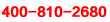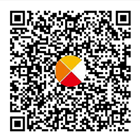# 北京小學四年級數學下冊7單元檢測題

2019-04-25 15:29:32 　來源：網絡整理

北京小學四年級數學下冊7單元檢測題我們努力學習的目的為什么呢？就是一定要掌握課本知識點，培養具有運用知識解決問題的能力。下面就是小編為大家帶來的北京小學四年級數學下冊7單元檢測題，希望可以幫助到大家。

想要了解【小學數學試題】的相關資料，請點擊加入【愛智康小學交流福利群】，并直接向管理員“小康康”索取！愛智康小學交流福利群會不定期免費發放學習資料，小學政策等相關消息，請持續關注！

一、“認真細致”填一填 。

1、35×102=35×100+□×□，這里運用了()律，這一運算定律用字母abc表示是(×()=+)。

2、35×16=35×○×○,這里運用了()律，結果是()。

3、在()里填上合適的數。

(30+2)×15=()×15+()×();

()×()-()×b=(85-a)×b ;

(17+)×(5)=()×5+5×3;

b×a+25×()=(+25)×b;

69×25×8=69 ×(×)。

4、橫著看，在得數相同的兩個算式后面的()內畫“√”錯的畫“×”。

(1)(64+36)×88和64×88+36×88。( √×)

(2)75×39+75和(39+1)×75。( √× )

(3)45×12和45×2×10。(×√× )

(4)40×50+50×90和40×(50+90)。( √× )

5、在里()填上“>”“<”或“=”。

29×30+30( ><= )30×30

74×56( ><= )65×47

125×16( ><= )125×8×8

47×99( ><= )47×100-1

二、“仔細推敲”判一判。

1、乘法分配律能使所有的乘法算式簡便。 ( √× )

2、102×98=(100+2)×98這里運用了乘法的分配律。 ( √× )

3、36×25=(9×4)×25=9+4×25。 ( √× )

4、125×17×8=125×8×17這里只運用了乘法結合律。 ( √× )

5、(40+7)×11=40+7×11。 ( √× )

三、1、直接寫出得數。

70×13=250×4=0÷280=456-199=100÷20=157+198=32×30=480÷16=

2、下面各題，怎樣算簡便就怎樣算。

45×99+45=103×23=28×25=24×73-24×127=125×25×64=25×(40-4)=

四、“走進生活”用一用。

1、學校買來67盒彩色粉筆和133盒白粉筆，每盒40枝，一共有多少支粉筆?

列式：答案

答：一共有支粉筆

2、水果店運來170筐蘋果，每筐45千克，售出一部分后，還剩80筐，賣出多少千克蘋果?

列式：答案

答：賣出千克蘋果。

3、一根繩子長168米，第一次用去57米，第二次用去43米，還剩多少米?

列式：答案

答：還剩米。

4、張華在新華書店買了2本《故事大王》和3盒磁帶，共用去42元，每盒磁帶8元，那么每本《故事大王》多少元?

列式： 答案

答：每本《故事大王》元。

5、花圃里有牡丹花84棵，月季花比牡丹花的3倍多12棵，月季花比牡丹花多多少棵?

列式：答案

答：月季花比牡丹花多朵。

小編推薦：

以上就是小編特意為大家整理的北京小學四年級數學下冊7單元檢測題希望對有需要的同學提供幫助，大家如果在學習中有什么疑問，歡迎撥打愛智康免費電話：!那里有專業的老師為大家解答。QQ掃一掃您將獲得群內福利家長互動海量資料同步免費視頻課程了解最新信息及時解讀資訊你可能感興趣的文章立即咨詢1對1在線課程*學而思愛智康會在24小時內與您取得電話聯系資訊動態熱門課程介紹資料領取學習相關10月13日初中入學規劃KET/PET備考

• 全國課程在線咨詢
• 咨詢熱線：4000-121-121
• 掃描注冊有禮

• 讓學習更有效